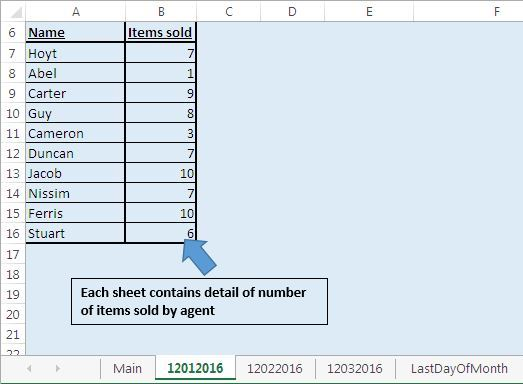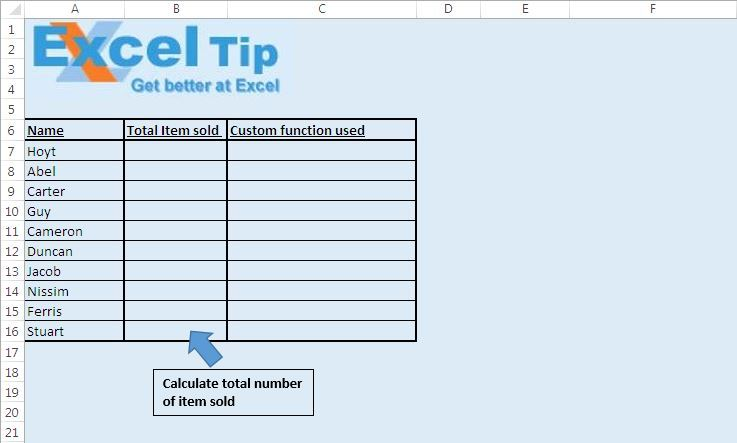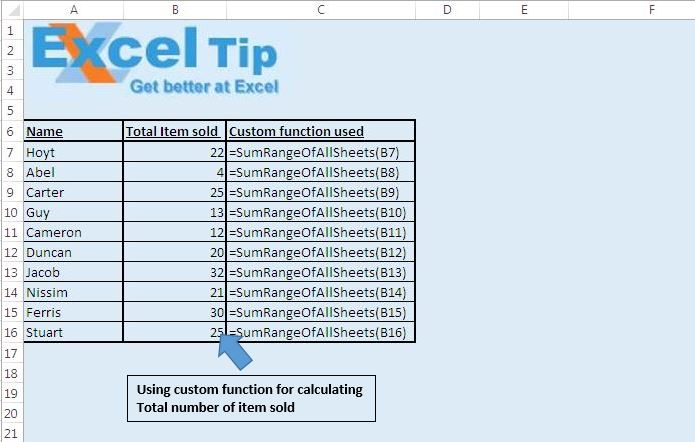# Add cells in a range from all worksheets in a workbook using VBA in Microsoft Excel

In this article, we will create a custom function to calculate the sum of cells in the defined range for all worksheets in a workbook.

Raw data for this example consists of multiple sheets. Each sheet contains data of the sales team, which includes sales person's name and number of items sold by him on the date specified by sheet name.

Sales data is present in the range A6:B16 in same sequence on all the worksheets.On the “Main” sheet, we want the total number of sales done by different sales persons. To calculate the total number of sales, we have to add the number of sales from all the sheets in the workbook.We have used custom function “SumRangeOfAllSheets” to calculate the total number of sales made by different sales persons. Custom function “SumRangeOfAllSheets” takes the range as input.Logic explanation

We have created custom function “SumRangeOfAllSheets” to calculate the sum of values in the cells in the defined range for all the worksheets in the workbook. Custom function “SumRangeOfAllSheets” takes the range as input. This function adds values in the cells of the defined range of all the worksheets.

Code explanation

WorksheetFunction.Sum

WorksheetFunction object is used to access Excel sheet functions from Visual Basics. We have used the SUM function of Excel sheet to add the values in the range.

Above code is used to return the address of the specified range by “InputRange” range object.

```
Option Explicit

Function SumRangeOfAllSheets(InputRange As Range)

'Declaring variables
Dim i As Integer
Dim Total As Long

'Looping through the sheets in the worksheets collection starting from second sheet
For i = 2 To Worksheets.Count

'Calculating the sum for specified range of all the sheets
Next

SumRangeOfAllSheets = Total

End Function

```